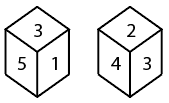Question 4

# Two different positions of the same dice are shown. Which number will be at the top if 6 is at the bottom?Solution

From the given image,

In the dice 1- 1 and 5 are the adjacent face of the 3.

In the dice 2- 2 and 4 are the adjacent face of the 3.

When we will overlap these two dice, then we can conclude that the opposite face of 3 will be 6.

Hence the opposite face of 6 will be 3.

• Free SSC Study Material - 18000 Questions
• 230+ SSC previous papers with solutions PDF